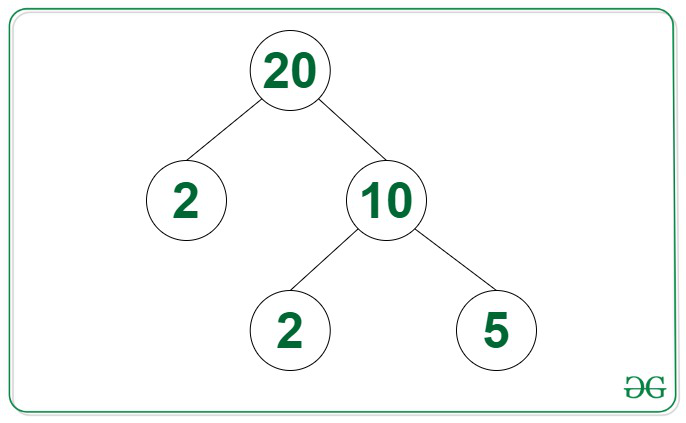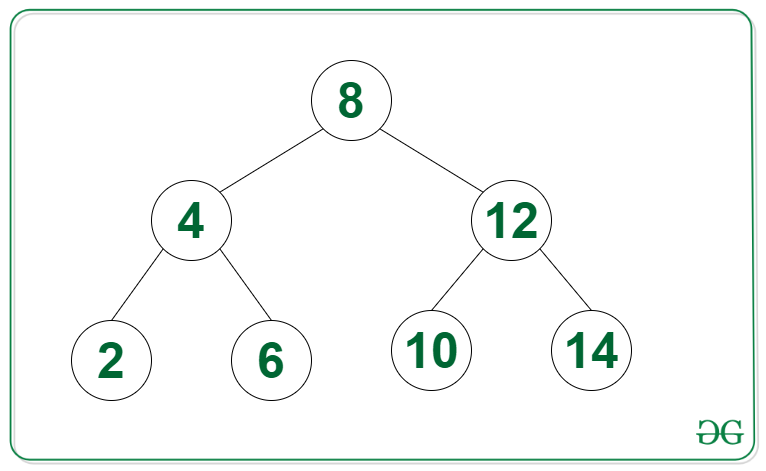Open in App
Not now

# Count of nodes in a binary tree having their nodes in range [L, R]

• Last Updated : 29 Jan, 2023

Given a Binary Tree consisting of N nodes and two positive integers L and R, the task is to find the count of nodes having their value in the range [L, R].

Examples:

Input: Tree in the image below, L = 4, R = 15Output: 2
Explanation: The nodes in the given Tree that lies in the range [4, 15] are {5, 10}.

Input: Tree in the image below, L = 8, R = 20Output: 4

Approach: The given problem can be solved by performing any Tree Traversal and maintaining the count of nodes having their values in the range [L, R]. This article uses a DFS traversal.

Below is the implementation of the above approach:

## C++

 `// C++ program for the above approach` `#include ` `using` `namespace` `std;`   `// Class for node of the Tree` `class` `Node {` `public``:` `    ``int` `val;` `    ``Node *left, *right;` `};`   `// Function to create a new Binary node` `Node* newNode(``int` `item)` `{` `    ``Node* temp = ``new` `Node();` `    ``temp->val = item;` `    ``temp->left = temp->right = NULL;`   `    ``// Return the newly created node` `    ``return` `temp;` `}`   `// Function to find the count of` `// nodes in the given tree with` `// their value in the range [1, N]` `int` `countRange(Node* root, ``int` `low,` `               ``int` `high, ``int` `count)` `{` `    ``int` `val = 0;`   `    ``// If root exists` `    ``if` `(root != NULL) {`   `        ``val += root->val >= low` `                       ``&& root->val <= high` `                   ``? 1` `                   ``: 0;` `    ``}`   `    ``// Otherwise return` `    ``else` `{` `        ``return` `0;` `    ``}`   `    ``// Add count of current node,` `    ``// count in left subtree, and` `    ``// count in the right subtree` `    ``count = val` `            ``+ countRange(root->left,` `                         ``low, high, count)` `            ``+ countRange(root->right,` `                         ``low, high, count);`   `    ``// Return Answer` `    ``return` `count;` `}`   `// Driver Code` `int` `main()` `{` `    ``Node* root = NULL;` `    ``root = newNode(20);` `    ``root->left = newNode(2);` `    ``root->right = newNode(10);` `    ``root->right->left = newNode(2);` `    ``root->right->right = newNode(5);`   `    ``int` `L = 4, R = 15;` `    ``cout << countRange(root, L, R, 0);`   `    ``return` `0;` `}`

## Java

 `// Java program for the above approach` `import` `java.util.*;` `class` `GFG{`   `  ``// Class for node of the Tree` `  ``static` `class` `Node {`   `    ``int` `val;` `    ``Node left, right;` `  ``};`   `  ``// Function to create a new Binary node` `  ``static` `Node newNode(``int` `item)` `  ``{` `    ``Node temp = ``new` `Node();` `    ``temp.val = item;` `    ``temp.left = temp.right = ``null``;`   `    ``// Return the newly created node` `    ``return` `temp;` `  ``}`   `  ``// Function to find the count of` `  ``// nodes in the given tree with` `  ``// their value in the range [1, N]` `  ``static` `int` `countRange(Node root, ``int` `low,` `                        ``int` `high, ``int` `count)` `  ``{` `    ``int` `val = ``0``;`   `    ``// If root exists` `    ``if` `(root != ``null``) {`   `      ``val += root.val >= low` `        ``&& root.val <= high` `        ``? ``1` `        ``: ``0``;` `    ``}`   `    ``// Otherwise return` `    ``else` `{` `      ``return` `0``;` `    ``}`   `    ``// Add count of current node,` `    ``// count in left subtree, and` `    ``// count in the right subtree` `    ``count = val` `      ``+ countRange(root.left,` `                   ``low, high, count)` `      ``+ countRange(root.right,` `                   ``low, high, count);`   `    ``// Return Answer` `    ``return` `count;` `  ``}`   `  ``// Driver Code` `  ``public` `static` `void` `main(String[] args)` `  ``{` `    ``Node root = ``null``;` `    ``root = newNode(``20``);` `    ``root.left = newNode(``2``);` `    ``root.right = newNode(``10``);` `    ``root.right.left = newNode(``2``);` `    ``root.right.right = newNode(``5``);`   `    ``int` `L = ``4``, R = ``15``;` `    ``System.out.print(countRange(root, L, R, ``0``));` `  ``}` `}`   `// This code is contributed by shikhasingrajput`

## Python3

 `# Python program for the above approach`   `# Class for Node of the Tree` `class` `Node:` `    ``def` `__init__(``self``, val):` `        ``self``.val ``=` `val;` `        ``self``.left ``=` `None``;` `        ``self``.right ``=` `None``;`   `# Function to create a new Binary Node` `def` `newNode(item):` `    ``temp ``=` `Node(item);` `    `  `    ``# Return the newly created Node` `    ``return` `temp;`   `# Function to find the count of` `# Nodes in the given tree with` `# their value in the range [1, N]` `def` `countRange(root, low, high, count):` `    ``val ``=` `0``;`   `    ``# If root exists` `    ``if` `(root !``=` `None``):`   `        ``val ``+``=` `1` `if``(root.val >``=` `low ``and` `root.val <``=` `high) ``else` `0``;`     `    ``# Otherwise return` `    ``else``:` `        ``return` `0``;`   `    ``# Add count of current Node,` `    ``# count in left subtree, and` `    ``# count in the right subtree` `    ``count ``=` `val ``+` `countRange(root.left, low, high, count) ``+` `countRange(root.right, low, high, count);`   `    ``# Return Answer` `    ``return` `count;`     `# Driver Code` `if` `__name__ ``=``=` `'__main__'``:` `    ``root ``=` `None``;` `    ``root ``=` `newNode(``20``);` `    ``root.left ``=` `newNode(``2``);` `    ``root.right ``=` `newNode(``10``);` `    ``root.right.left ``=` `newNode(``2``);` `    ``root.right.right ``=` `newNode(``5``);`   `    ``L ``=` `4``;` `    ``R ``=` `15``;` `    ``print``(countRange(root, L, R, ``0``));`   `# This code is contributed by 29AjayKumar`

## C#

 `// C# program for the above approach` `using` `System;`   `public` `class` `GFG{`   `  ``// Class for node of the Tree` `  ``class` `Node {`   `    ``public` `int` `val;` `    ``public` `Node left, right;` `  ``};`   `  ``// Function to create a new Binary node` `  ``static` `Node newNode(``int` `item)` `  ``{` `    ``Node temp = ``new` `Node();` `    ``temp.val = item;` `    ``temp.left = temp.right = ``null``;`   `    ``// Return the newly created node` `    ``return` `temp;` `  ``}`   `  ``// Function to find the count of` `  ``// nodes in the given tree with` `  ``// their value in the range [1, N]` `  ``static` `int` `countRange(Node root, ``int` `low,` `                        ``int` `high, ``int` `count)` `  ``{` `    ``int` `val = 0;`   `    ``// If root exists` `    ``if` `(root != ``null``) {`   `      ``val += root.val >= low` `        ``&& root.val <= high` `        ``? 1` `        ``: 0;` `    ``}`   `    ``// Otherwise return` `    ``else` `{` `      ``return` `0;` `    ``}`   `    ``// Add count of current node,` `    ``// count in left subtree, and` `    ``// count in the right subtree` `    ``count = val` `      ``+ countRange(root.left,` `                   ``low, high, count)` `      ``+ countRange(root.right,` `                   ``low, high, count);`   `    ``// Return Answer` `    ``return` `count;` `  ``}`   `  ``// Driver Code` `  ``public` `static` `void` `Main(String[] args)` `  ``{` `    ``Node root = ``null``;` `    ``root = newNode(20);` `    ``root.left = newNode(2);` `    ``root.right = newNode(10);` `    ``root.right.left = newNode(2);` `    ``root.right.right = newNode(5);`   `    ``int` `L = 4, R = 15;` `    ``Console.Write(countRange(root, L, R, 0));` `  ``}` `}`   `// This code is contributed by shikhasingrajput`

## Javascript

 ``

Output

`2`

Time Complexity : O(n), where n is the number of nodes in the tree
Auxiliary Space: O(h), where h is the height of the tree.

Another Approach(Iterative):
The given problem can be solved by using the Level Order Traversal

. Follow the steps below to solve the problem:

1) Create a queue(q) and initialize count variable with 0, and store the nodes in q along wise level order and iterate for next level.
2) Perform level order traversal and check if current node value lie in range then increment the count variable.
3) After completing the above steps, return count variable.

Below is the implementation of above approach:

## C++

 `// C++ program for the above approach` `#include` `using` `namespace` `std;`   `// struct of binary tree node` `struct` `Node{` `    ``int` `data;` `    ``Node* left;` `    ``Node* right;` `};`   `// function to create a new node` `Node* newNode(``int` `data){` `    ``Node* temp = ``new` `Node();` `    ``temp->data = data;` `    ``temp->left = temp->right = NULL;` `    ``return` `temp;` `}`   `// Function to find the count of` `// nodes in the given tree with` `// their value in the range [1, N]` `int` `countRange(Node* root, ``int` `low, ``int` `high){` `    ``int` `count = 0;` `    `  `    ``// if root exists` `    ``if``(root == NULL) ``return` `count;` `    ``queue q;` `    ``q.push(root);` `    ``while``(!q.empty()){` `        ``Node* temp = q.front();` `        ``q.pop();` `        ``if``(temp->data <= high && temp->data >= low){` `            ``count++;` `        ``}` `        ``if``(temp->left != NULL) q.push(temp->left);` `        ``if``(temp->right != NULL) q.push(temp->right);` `    ``}` `    ``return` `count;` `}`   `//driver code` `int` `main(){` `    ``Node *root = newNode(20);` `    ``root->left = newNode(2);` `    ``root->right = newNode(10);` `    ``root->right->left = newNode(2);` `    ``root->right->right = newNode(5);` ` `  `    ``int` `L = 4, R = 15;` `    ``cout << countRange(root, L, R);` ` `  `    ``return` `0;` `}`   `// This code is contributed by Kirti Agarwal(kirtiagarwal23121999)`

Output

`2`

Time Complexity: O(N) where N is the number of nodes in given binary tree.
Auxiliary Space: O(N) due to queue data structure.

My Personal Notes arrow_drop_up
Related Articles